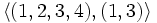# Generating sets for subgroups of symmetric group:S4

This article gives specific information, namely, generating sets for subgroups, about a particular group, namely: symmetric group:S4.
View generating sets for subgroups of particular groups | View other specific information about symmetric group:S4

This article provides summary information on various choices of generating set for subgroups of symmetric group:S4. It builds on basic information available at element structure of symmetric group:S4 and subgroup structure of symmetric group:S4.

## Probability of generation

The rule is as follows. Given$k$ (not necessarily distinct) elements picked uniformly at random and independently of each other from a finite group$G$, the probability that they all live in a fixed subgroup of index$d$ is$1/d^k$.

Using this and a form of Mobius inversion on the subgroup lattice, it is possible to compute the probability that they generate a fixed subgroup of index$d$ (we basically need to subtract off probabilities for smaller subgroups).

### Generated by one element

Here, a single element is picked uniformly at random from the group.

Automorphism class of subgroups Representative Isomorphism class Order of subgroups Index of subgroups Probability that an element is in a fixed subgroup of this automorphism class(= reciprocal of index) Probability that an element generates a fixed subgroup of this automorphism class (equal to preceding column if the subgroup is cyclic, zero otherwise) Size of automorphism class Probability that the element generates a subgroup in this automorphism class
trivial subgroup$\{ () \}$ trivial group 1 24 1/24 1/24 1 1/24
S2 in S4$\{ (), (1,2) \}$ cyclic group:Z2 2 12 1/12 1/24 6 1/4
subgroup generated by double transposition in S4$\{ (), (1,2)(3,4) \}$ cyclic group:Z2 2 12 1/12 1/24 3 1/8
A3 in S4$\{ (), (1,2,3), (1,3,2) \}$ cyclic group:Z3 3 8 1/8 1/12 4 1/3
Z4 in S4$\langle (1,2,3,4) \rangle$ cyclic group:Z4 4 6 1/6 1/12 3 1/4
normal Klein four-subgroup of S4$\{ (), (1,2)(3,4), (1,3)(2,4), (1,4)(2,3) \}$ Klein four-group 4 6 1/6 0 1 0
non-normal Klein four-subgroups of S4$\langle (1,2), (3,4) \rangle$ Klein four-group 4 6 1/6 0 3 0
S3 in S4$\langle (1,2,3), (1,2) \rangle$ symmetric group:S3 6 4 1/4 0 4 0
D8 in S4$\langle (1,2,3,4), (1,3) \rangle$ dihedral group:D8 8 3 1/3 0 3 0
A4 in S4$\langle (1,2,3), (1,2)(3,4) \rangle$ alternating group:A4 12 2 1/2 0 1 0
whole group$\langle (1,2,3,4), (1,2) \rangle$ symmetric group:S4 24 1 1 0 1 0
Total (11 rows) -- -- -- -- -- -- 30 1

### Generated by two independent possibly equal elements

Here, two elements are picked uniformly at random from the group, independent of each other. They could be equal.

Automorphism class of subgroups Representative Isomorphism class Order of subgroups Index of subgroups Probability that the elements are in a fixed subgroup of this automorphism class(= reciprocal square of index) Conditional probability that they generate the subgroup given they're in it (depends only on its isomorphism class) Probability that the elements generates a fixed subgroup of this automorphism class (obtained by multiplying preceding two columns) Size of automorphism class Probability that the elements generate a subgroup in this automorphism class
trivial subgroup$\{ () \}$ trivial group 1 24 1/576 1 1/576 1 1/576
S2 in S4$\{ (), (1,2) \}$ cyclic group:Z2 2 12 1/144 3/4 1/192 6 1/32
subgroup generated by double transposition in S4$\{ (), (1,2)(3,4) \}$ cyclic group:Z2 2 12 1/144 3/4 1/192 3 1/64
A3 in S4$\{ (), (1,2,3), (1,3,2) \}$ cyclic group:Z3 3 8 1/64 8/9 1/72 4 1/18
Z4 in S4$\langle (1,2,3,4) \rangle$ cyclic group:Z4 4 6 1/36 3/4 1/48 3 1/16
normal Klein four-subgroup of S4$\{ (), (1,2)(3,4),$$(1,3)(2,4), (1,4)(2,3) \}$
Klein four-group 4 6 1/36 3/8 1/96 1 1/96
non-normal Klein four-subgroups of S4$\langle (1,2), (3,4) \rangle$ Klein four-group 4 6 1/36 3/8 1/96 3 1/32
S3 in S4$\langle (1,2,3), (1,2) \rangle$ symmetric group:S3 6 4 1/16 1/2 1/32 4 1/8
D8 in S4$\langle (1,2,3,4), (1,3) \rangle$ dihedral group:D8 8 3 1/9 3/8 1/24 3 1/8
A4 in S4$\langle (1,2,3), (1,2)(3,4) \rangle$ alternating group:A4 12 2 1/4 2/3 1/6 1 1/6
whole group$\langle (1,2,3,4), (1,2) \rangle$ symmetric group:S4 24 1 1 3/8 3/8 1 3/8
Total (11 rows) -- -- -- -- -- -- -- -- 1

## Small generating sets for subgroups

We know that the subgroup rank of the symmetric group of degree four is 2, i.e., all its subgroups are 2-generated groups. On the other hand, we also know that it is possible to come up with small generating sets that are not of minimum size. Some information on this is included below.

For brevity, we do not include all subsets of interest but describe our subsets by means of generic descriptors.

Subset Size Number of such subsets Subgroup it generates Is it a generating set of minimum size for the subgroup it generates? (if Yes, size at most 2) Is it a minimal generating set for the subgroup it generates? (if Yes, size at most 3) Is it a Jerrum-reduced generating set? (if Yes, size at most 3) Is it a Sims-reduced generating set? (if Yes, size at most 6)$\{ \}$ 0 1 trivial Yes Yes Yes Yes$\{ (i,j) \}$ 1 6 S2 in S4 Yes Yes Yes Yes$\{ (i,j,k) \}$ 1 8 A3 in S4 Yes Yes Yes Yes$\{ (i,j)(k,l) \}$ 1 3 subgroup generated by double transposition in S4 Yes Yes Yes Yes$\{ (i,j,k,l) \}$ 1 6 Z4 in S4 Yes Yes Yes Yes$\{ (i,j), (k,l) \}$, all distinct 2 3 non-normal V4 in S4 Yes Yes Yes Yes$\{ (i,j), (i,k) \}$,$i < j < k$ 2 4 S3 in S4 Yes Yes No Yes$\{ (i,j), (j,k) \}$,$i < j < k$ 2 4 S3 in S4 Yes Yes Yes Yes$\{ (i,k), (j,k) \}$,$i < j < k$ 2 4 S3 in S4 Yes Yes Yes Yes

(More rows need to be filled in)